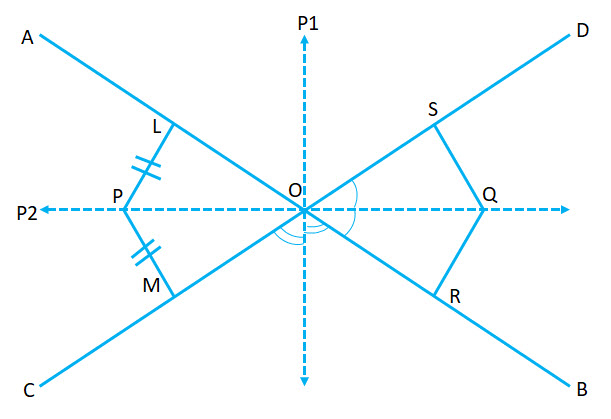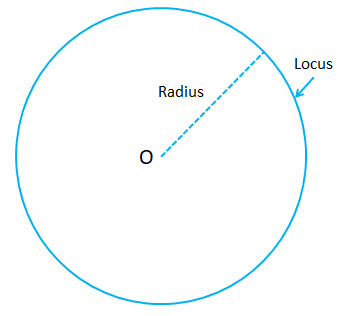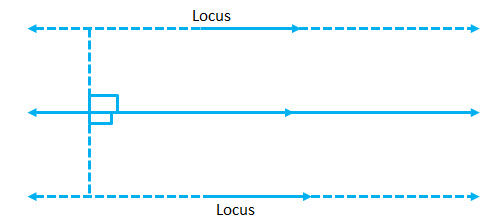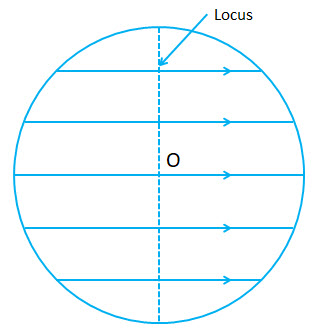Definition:

In geometry, a locus (plural: loci) (Latin word for “place”, “location”) is a set of all points (commonly, a line, a line segment, a curve or a surface), whose location satisfies or is determined by one or more specified conditions.

In another terms…Locus can be defined as the path traced by a point, which moves so as to satisfy certain given conditions such as equidistant from two given lines, equidistant from a given point etc.

Theorems based on symmetry:

 Theorem 2: The locus of a point equidistant from two intersecting lines is the bisector of the angles between the lines.Given: Two straight lines$AB \ and \ CD$ intersecting at$O$ . Point$P$ is such that it is equidistant from$AB \ and \ CD$ (the two given lines)

To Prove: Locus of$P$ is the bisector of$\angle AOC$

(i)$\Rightarrow P$ lies on the bisector of$\angle AOC$

(ii)$\Rightarrow$ Each point on the bisector of$\angle AOC$ is equidistant from$AB \ and \ CD$

Proof: Consider$\triangle POL$ and$\triangle POM$

(i)$PL = PM$ (Given)$\angle PLO = \angle PMO$ (right angles triangle)$PO$ is common

Therefore$\triangle POL \cong \triangle POM$$\Rightarrow \angle POL = \angle POM$

Therefore$P$ lies on the angle bisector of$\angle AOC$

(ii) Conversely, if$Q$ be any point on the angle bisector$OP$

Consider$\triangle QOS \ and \ \triangle QOR$$\angle SOQ = \angle ROQ$ (given)$OQ$ is common$\angle SQO = \angle RQO$

Therefore$\triangle QOS \cong \triangle QOR$

Hence$QS = QR$$\Rightarrow Q \ is \ equidistant \ from \ AB \ and \ CD$

 Theorem 3: The set of points equidistant from two points is a perpendicular bisector to the line segment connecting the two points.Given: Two fixed points$A \ and \ B$.$P$ is a point equidistant from$A \ and \ B \ i.e. \ PA = PB$ at all times.

To Prove: Locus of moving point$P$ is perpendicular bisector of line$AB$

(i)$P$ lies on perpendicular bisector of$AB$ and conversely

(ii) Every point on this perpendicular bisector is equidistant from points$A \ and \ B$

Proof:

(i)   Consider$\triangle AOP \ and \ \triangle BOP$$PA=PB$ (Given)$AO = OB$ (Given)$PO$ is common

Therefore$\triangle AOP \cong \triangle BOP$ (S.S.S postulate)

Therefore corresponding angles are equal

Hence$\angle AOP = \angle BOP$

Since$\angle AOP + \angle BOP = 180 \Rightarrow \angle AOP = 90^o$

Hence proved that$P$ lies on perpendicular bisector of$AB$

(ii)  Given$PO$ is the perpendicular bisector of$AB$

Consider$\triangle AOQ \ and \ \triangle BOQ$$AO=OB$$\angle AOQ = \angle BOQ$$OQ$ is common

Therefore$\triangle AOQ \cong \triangle BOQ$

Hence$AQ=BQ$

Therefore proved that every point on a perpendicular bisector is equidistant from fixed points$A \ and \ B$.

Notes:

 The locus of a point in a plane at a fixed distance from a given point is the circumference of a circle with the fixed given point as the center of the circle and the distance as the radius.The locus of a point equidistant from two given parallel lines is a line parallel to the given lines and is midway between them.The locus of a point, which is at a given distance from a given line, is a pair of lines parallel to the given line and at the given distance from it.The locus of all mid-points of all equal chords, in a circle, is the circumference of the circle concentric with the given circle and having radius equal to the distance of equal chords from the center.The locus of mid-point of all parallel chords in a circle is the diameter of the circle which is perpendicular to the given parallel chords.The locus of a point equidistant from two concentric circles is the circumference of the circle concentric with the given circle and midway between them.